Get instant live expert help with Excel or Google Sheets“My Excelchat expert helped me in less than 20 minutes, saving me what would have been 5 hours of work!”

#### Post your problem and you’ll get expert help in seconds.

Your message must be at least 40 characters
Our professional experts are available now. Your privacy is guaranteed.

# Learn How to Extract Random Data in an Excel Spreadsheet

Excel allows us to extract random data from the list using the RAND, INDEX and RANK functions. This step by step tutorial will assist all levels of Excel users to get the random names from the list and to create a random team of three members.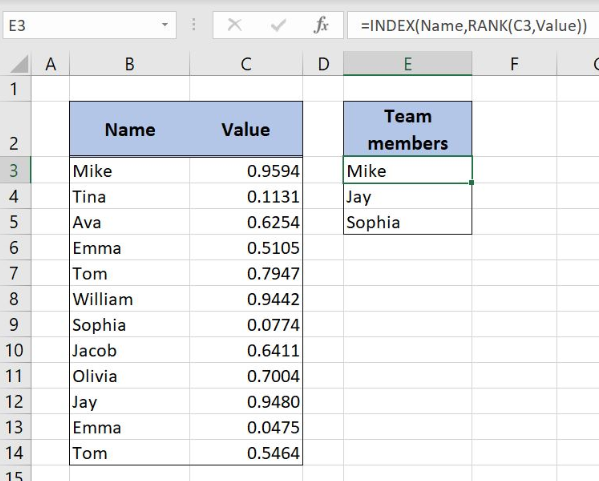Figure 1. Get the random team members from the name list

## Syntax of the RAND Formula

The generic formula for the RAND function is:

`=RAND()`

The function returns a random decimal number between 0 and 1 and has no parameters.

## Syntax of the INDEX Formula

The generic formula for the INDEX function is:

`=INDEX(array, row_num, column_num)`

The parameters of the INDEX function are:

• array – a range of cells where we want to get a data
• row_num – a number of a row in the array for which we want to get a value
• column_num – a column in the array which returns a value.

## Syntax of the RANK Formula

The generic formula for the RANK function is:

`=RANK(number, ref, [order])`

The parameters of the RANK function are:

• number – a number which we want to rank
• ref – a list of numbers for ranking
• [order] – 1 for ascending or 0 for descending order. This parameter is optional, if it’s omitted, the default order is descending

## Setting up Our Data to Extract Random Data from the Name List

Our table consists of 2 columns: “Name” (column B) and “Value” (column C). In column “Value” will be placed random numbers from 0 to 1 that will help us in the calculation. The second table has column “Team members” (column E) where we will extract the names from column B.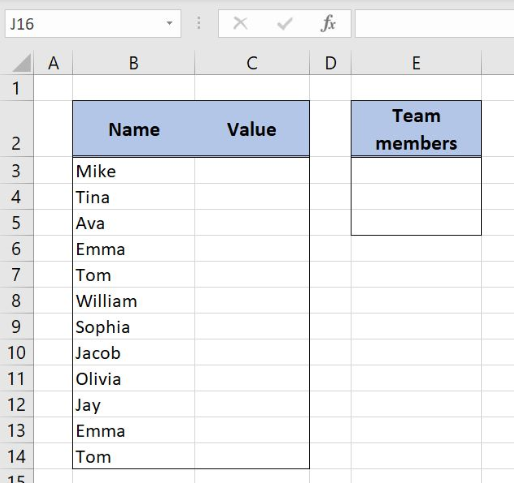Figure 2. Data for the example

## Get Random Names from the Table with RAND, INDEX and RANK Functions

We want to get the random names from column B and to place the results in the column E. In order to make the formula more clear, we will create a named range Name for cell range B3:B14 and a named range Value for the cell range C3:C14.

To create a named range we should follow the steps:

• Select the cell range that should be named
• Click on the name box in Excel
• Write the name for the cell range and press enter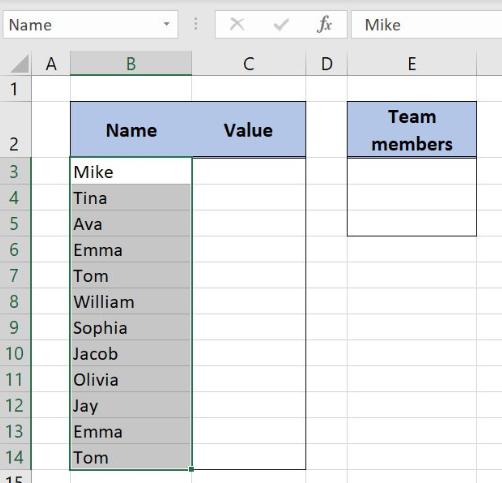Figure 3. Creating a named range Name for column “Name”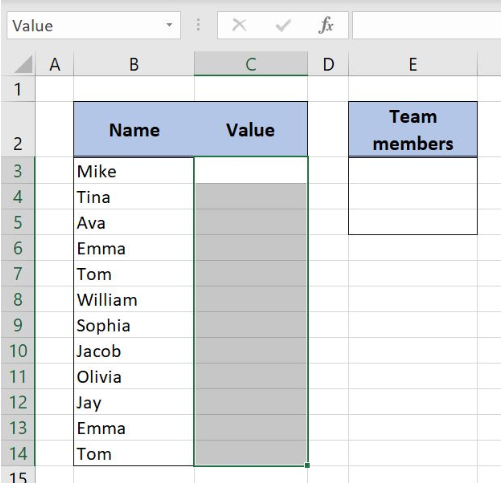Figure 4. Creating a named range Value for column “Value”

The formula in Column C looks like:

`=RAND()`

The RAND function gets the random value from 0 to 1. The randomized values are placed in column C and will help us to get the random names in column E.

To apply the formula, we need to follow these steps:

• Select cell C3 and click on it
• Insert the formula: `=RAND()`
• Press enter
• Drag the formula down to the other cells in the column by clicking and dragging the little “+” icon at the bottom-right of the cell.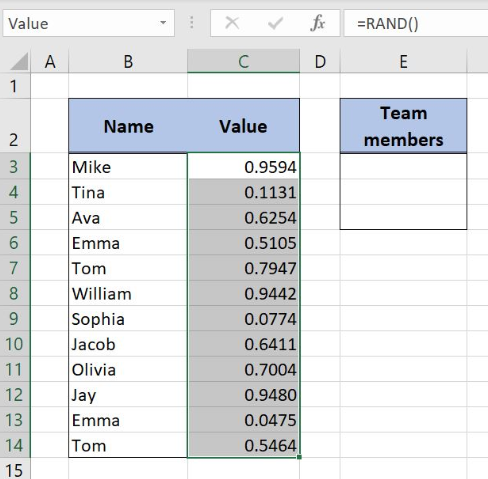Figure 5. Using the RAND formula to get a random value between 0 and 1

Now we can place the formula in the cell E3 to get the random team member:

`=INDEX(Name,RANK(C3,Value))`

The parameter array in the INDEX function is a named range Name while the row_num is the formula RANK(C3,Value). In the RANK function, the number is a cell C3 while the parameter ref is the named range Value.

To apply the formula, we need to follow these steps:

• Select cell E3 and click on it
• Insert the formula: `=INDEX(Name,RANK(C3,Value))`
• Press enter
• Drag the formula down to the other cells in the column by clicking and dragging the little “+” icon at the bottom-right of the cell.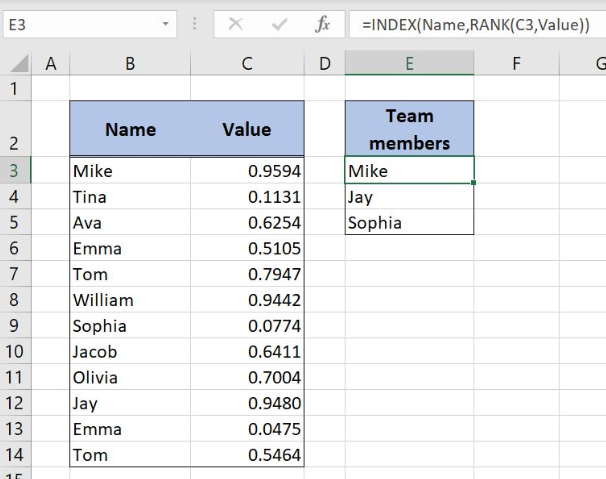Figure 6. Extract random data with RAND, INDEX and RANK functions

With RANK function we get the rank of the cell C3 in column C. Since the values are unique in the “Value” column the result from the RANK function is a row number input for the INDEX function. The RANK function result is number 1 and the INDEX formula retrieves the first entry in the named range Name. Final formula output is Mike, a random name from column B.

Most of the time, the problem you will need to solve will be more complex than a simple application of a formula or function. If you want to save hours of research and frustration, try our live Excelchat service! Our Excel Experts are available 24/7 to answer any Excel question you may have. We guarantee a connection within 30 seconds and a customized solution within 20 minutes.

Solution examplesI need help on index and match where the return is not correct
Solved by B. B. in 20 minsI have a database extract that is showing the same clients multiple times in the list. How can I write a formula in excel to look at the column with the client names and return only unique records on another sheet? I want to run this over several files so wanting to avoid using pivot tables which is how I would normal do this.
Solved by X. J. in 20 minsim creating a fantasy hockey model and trying to figure out how to rank if someone has played more than a certain number of games, and if they're certain position how to rank them accordingly
Solved by X. D. in 60 minsHi, I have created a drop down list and in another cell I have used an IF function which is dependent on the drop down list. However, when i change the selected option from the drop down list, the IF function cell does not update accordingly
Solved by C. C. in 17 minsI work shift work as do about 100 other guys. All I want to do is type in the days date (Or just open the sheet and it will have the days date) and it has on there who is working that day/night. Pretty simple I would think...And if I change the date to, let's say, a month down the road, it pulls in the guys working that day... That all...Thx
Solved by O. E. in 20 mins